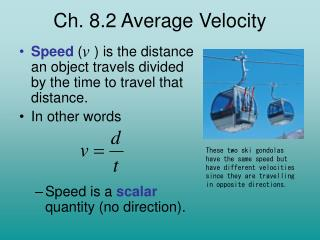DownloadDownload PresentationCh. 8.2 Average Velocity

# Ch. 8.2 Average Velocity

Télécharger la présentation## Ch. 8.2 Average Velocity

- - - - - - - - - - - - - - - - - - - - - - - - - - - E N D - - - - - - - - - - - - - - - - - - - - - - - - - - -
##### Presentation Transcript

1. Ch. 8.2 Average Velocity • Speed ( ) is the distance an object travels divided by the time to travel that distance. • In other words • Speed is a scalar quantity (no direction). These two ski gondolas have the same speed but have different velocities since they are travelling in opposite directions.

2. Velocity( ) is the displacement of an object divided by the time interval. • In other words • Velocity describes how fast an object’s • position is changing. • Velocity is a vector quantity (direction). • The units for velocity and speed are metres • per second (m/s).

3. Calculating the Slope of the Position-Time Graph • To learn more about velocity, we must now understand straight line graphs. • The slope of a graph is represented by • rise = change in y-axis • run = change in x-axis

4. 500 400 300 Position (m) 400 200 11 100 0 2 4 6 8 10 12 14 16 18 20 Time (s) Finding the Slope of a Line • Pick two points on the grid. 2. Make a triangle 3. 4. Units = metres/second So slope = 36.4 m/s

5. Position – Time Graph • On a position-time graph the slope is the change in position ( ) divided by the change in time ( ).

6. Example Which jogger’s motion has a greater slope? Which jogger is moving faster? What is the slope of each line? What is each jogger’s ?

7. Average Velocity • Usually, objects do not move at a perfectly constant speed. • For this reason, we often use “average velocity” in our calculations. • The slope of a position-time graph is the object’s average velocity ( ). • Average velocity is the rate of change in position for a time interval.

8. Remember , (average velocity), is a vector and we must therefore always include a direction. • If we were to discuss , (average speed), we do not need to include direction, because speed is a scalar.

9. On a position-time graph if “forward” is given a positive direction: • A positive slope means that the object’s average velocity is forward. • A negative slope means that the object’s average velocity is backwards. • Zero slope means the object’s average velocity is zero.

10. Step Four: Calculate answer. Step Three: Insert values into equation Calculating Average Velocity Example 1 The relationship between average velocity, displacement, and time is given by: What is the average velocity of a dog that takes 4.0 s to run forward 14 m? Solution Step One: Write out known values. Step Two: Write down equation.

11. Step Four: Calculate answer. Step Three: Insert values into equation Example 2 A boat travels 280 m East in a time of 120 s. What is the boat’s average velocity? Solution Step One: Write out known values. Step Two: Write down equation.

12. Multiply both sides by . The on the right cancels out leaving the equation: Calculating Displacement The formula for finding velocity can be rearranged so that you can solve for either displacement or time. You can solve for time by taking this equation and divide both sides by leaving the equation:

13. Step Four: Calculate answer. Step Three: Insert values into equation Example 3 What is the displacement of a bicycle that travels 8.0 m/s [N] for 15 s? Solution Step One: Write out known values. Step Two: Write down equation.

14. Example 4 Solve this problem on your own, using the same steps. 4. A person, originally at the starting line, runs west at 6.5 m/s. What is the runner’s displacement after 12 s? Answer: 78 m West

15. Calculating Time Use the last equation to solve the following: • How long would it take a cat walking north at 0.80 m/s to travel 12 m north? Solution: • A car is driving forward at 15 m/s. How long would it take this car to pass through an intersection that is 11 m long? Solution:

16. Converting between m/s and km/h • To convert from km/h to m/s • Change km to m: 1 km = 1000 m • Change h to s: 1 h = 3600 s • Therefore multiply by 1000 and divide by 3600 or • Divide the speed in km/h by 3.6 to obtain the speed in m/s. For example, convert 75 km/h to m/s.

17. Converting between m/s and km/h Try the following unit conversion problems yourself. 1. Convert 95 km/h to m/s. • 95 km X 1000m X 1hr 1hr 1km 3600s • A truck’s displacement is 45 km north after driving for 1.3 hours. What was the truck’s average velocity in km/h & m/s? • What is the displacement of an airplane flying 480 km/h [E] during a 5.0 min time interval? = 26 m/s Vav = 45km 1.3hr = 35 km/h N or 9.6 m/s N 40 km [E] or 40, 000 m [E] d = 480 km X .083 hr hr Take the Section 8.2 Quiz